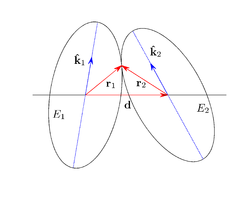# Distance of closest approach of ellipses and ellipsoids

﻿
Distance of closest approach of ellipses and ellipsoids

The distance of closest approach of two objects is the distance between their centers when they are externally tangent. The objects may be geometric shapes or physical particles with well defined boundaries. The distance of closest approach is sometimes referred to as the contact distance.

For the simplest objects, spheres, the distance of closest approach is simply the sum of their radii. For non-spherical objects, the distance of closest approach is a function of the orientation of the objects, and its calculation can be difficult. The maximum packing density of hard particles, an important problem of ongoing interest, depends on their distance of closest approach.

The interactions of particles typically depend on their separation, and the distance of closest approach plays an important role in determining the behavior of condensed matter systems.

The excluded volume of particles (the volume excluded to the centers of other particles due to the presence of one) is a key parameter in such descriptions,; the distance of closest approach is required to calculate the excluded volume. The excluded volume for identical spheres is just four times the volume of one sphere. For other anisotropic objects, the excluded volume depends on orientation, and its calculation can be surprising difficult. The simplest shapes after spheres are ellipses and ellipsoids; these have received considerable attention, yet their excluded volume is not known. Vieillard Baron was able to provide an overlap criterion for two ellipses. His results were useful for computer simulations of hard particle systems and for packing problems using Monte Carlo simulations.Two externally tangent ellipses

The one anisotropic shape whose excluded volume can be expressed analytically is the spherocylinder; the solution of this problem is a classic work by Onsager. The problem was tackled by considering the distance between two line segments, which are the center lines of the capped cylinders. Results for other shapes are not readily available. The orientation dependence of the distance of closest approach has surprising consequences. Systems of hard particles, whose interactions are only entropic, can become ordered. Hard spherocylinders form not only orientationally ordered nematic, but also positionally ordered smectic phases. Here, the system gives up some (orientational and even positional) disorder to gain disorder and entropy elsewhere.

## Distance of closest approach of two ellipses

Vieillard Baron first investigated this problem, although he did not obtain a result for the distance of closest approach, he derived the overlap criterion for two ellipses. His results were useful for the study of the phase behavior of hard particles and for the packing problem using Monte Carlo simulations. Although overlap criteria have been developed, analytic solutions for the distance of closest approach and the location of the point of contact have only recently become available. The details of the calculations are provided in Ref. The Fortran 90 subroutine is provided in Ref.

### The method

The procedure consists of three steps:

1. Transformation of the two tangent ellipses E1 and E2, whose centers are joined by the vector d, into a circle C1' and an ellipse E2', whose centers are joined by the vector d'. The circle C1' and the ellipse E2' remain tangent after the transformation.
2. Determination of the distance d' of closest approach of C1' and E2' analytically. It requires the appropriate solution of a quartic equation. The normal n' is calculated.
3. Determination of the distance d of closest approach and the location of the point of contact of E1 and E2 by the inverse transformations of the vectors d' and n'.

Input:

• lengths of the semiaxes a1,b1,a2,b2,
• unit vectors k1,k2 along major axes of both ellipses, and
• unit vector d joining the centers of the two ellipses.

Output:

• distance d between the centers when the ellipses E1 and E2 are externally tangent, and
• location of point of contact in terms of k1,k2.

## Distance of closest approach of two ellipsoids

Consider two ellipsoids, each with a given shape and orientation, whose centers are on a line with given direction. We wish to determine the distance between centers when the ellipsoids are in point contact externally. This distance of closest approach is a function of the shapes of the ellipsoids and their orientation. There is no analytic solution for this problem, since solving for the distance requires the solution of a sixth order polynomial equation. Here an algorithm is developed to determine this distance, based on the analytic results for the distance of closest approach of ellipses in 2D, which can be implemented numerically. Details are given in publications. Subroutines are provided in two formats: Fortran90  and C.

### Method

The algorithm consists of three steps.

1. Constructing a plane containing the line joining the centers of the two ellipsoids, and finding the equations of the ellipses formed by the intersection of this plane and the ellipsoids.
2. Determining the distance of closest approach of the ellipses; that is the distance between the centers of the ellipses when they are in point contact externally.
3. Rotating the plane until the distance of closest approach of the ellipses is a maximum. The distance of closest approach of the ellipsoids is this maximum distance.

Wikimedia Foundation. 2010.